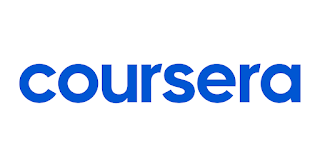Exploratory Data Analysis complete course is currently being offered by Johns Hopkins University through Coursera platform and is being taught by Brian Caffo, Jeff Leek and Roger D. Peng

Learning Outcomes

Understand analytic graphics and the base plotting system in R

- Use advanced graphing systems such as the Lattice system

- Make graphical displays of very high dimensional data

- Apply cluster analysis techniques to locate patterns in data

Skills You Will Gain

• Cluster Analysis
• Ggplot2
• R Programming
• Exploratory Data Analysis

Also Check: How to Apply for Coursera Financial AidExploratory Data Analysis Week 1 Quiz Answers - Coursera!

Question 1. Which of the following is a principle of analytic graphics?

Make judicious use of color in your scatterplots (NO)

Don’t plot more than two variables at at time (NO)

Show box plots (univariate summaries) (NO)

Only do what your tools allow you to do (NO)

Show comparisons

Question 2. What is the role of exploratory graphs in data analysis?

• Axes, legends, and other details are clean and exactly detailed.
• They are typically made very quickly.
• Only a few are constructed.
• They are made for formal presentations.

Question 3. Which of the following is true about the base plotting system?

• The system is most useful for conditioning plots
• Plots are created and annotated with separate functions
• Plots are typically created with a single function call
• Margins and spacings are adjusted automatically depending on the type of plot and the data

Question 4. Which of the following is an example of a valid graphics device in R?

• The keyboard
• A file folder
• A Microsoft Word document
• A PNG file
• A socket connection

Question 5. Which of the following is an example of a vector graphics device in R?

• TIFF
• GIF
• Postscript
• PNG
• JPEG

Question 6. Bitmapped file formats can be most useful for

• Scatterplots with many many points
• Plots that require animation or interactivity
• Plots that are not scaled to a specific resolution
• Plots that may need to be resized

Question 7. Which of the following functions is typically used to add elements to a plot in the base graphics system?

• hist()
• boxplot()
• plot()
• text()

Question 8. Which function opens the screen graphics device for the Mac?

• quartz()
• bitmap()
• pdf()
• png()

Question 9. What does the ‘pch’ option to par() control?

• the plotting symbol/character in the base graphics system
• the line width in the base graphics system
• the orientation of the axis labels on the plot
• the size of the plotting symbol in a scatterplot

Question 10. If I want to save a plot to a PDF file, which of the following is a correct way of doing that?

• Construct the plot on the screen device and then copy it to a PDF file with dev.copy2pdf()
• Construct the plot on the PNG device with png(), then copy it to a PDF with dev.copy2pdf().
• Open the screen device with quartz(), construct the plot, and then close the device with dev.off().
• Open the PostScript device with postscript(), construct the plot, then close the device with dev.off().
Alternate Questions

1. What does the ‘pch’ option to par() control?

• the size of the plotting symbol in a scatterplot
• the plotting symbol/character in the base graphics system
• the orientation of the axis labels on the plot
• the line width in the base graphics system

2. Which function opens the screen graphics device on Windows?

postscript()

windows()

jpeg()

xfig()

3. Which of the following is a principle of analytic graphics?

• Show box plots (univariate summaries)
• Only do what your tools allow you to do
• Show causality, mechanism, explanation
• Make judicious use of color in your scatterplots
• Don’t plot more than two variables at at time

4. Which of the following is an example of a valid graphics device in R?

A file folder (NO)

The computer screen

A Microsoft Word document (NO)

A socket connection (NO)

The keyboard (NO)

5. Which of the following is an example of a vector graphics device in R?

GIF

TIFF

SVG

PNG

JPEG

6. Which of the following functions is typically used to add elements to a plot in the base graphics system?

hist()

plot()

points()

boxplot()